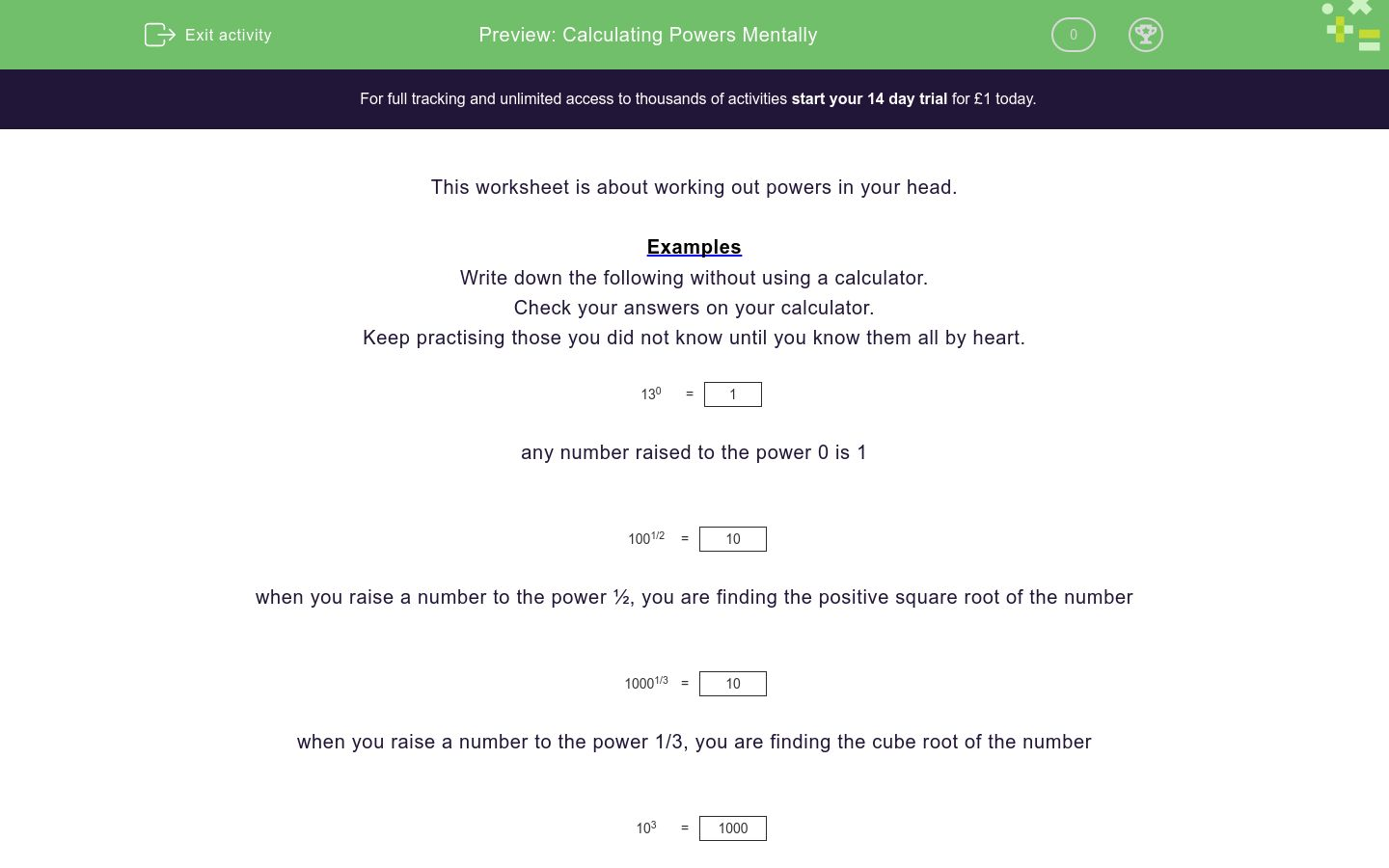# Calculating Powers Mentally

In this worksheet, students practise calculating powers mentally.Key stage:  KS 4

Curriculum topic:  Number

Curriculum subtopic:  Calculate with Roots and Integer (Fractional) Indice

Difficulty level:### QUESTION 1 of 10

Examples

Write down the following without using a calculator.
Keep practising those you did not know until you know them all by heart.

130 =
 1

any number raised to the power 0 is 1

1001/2 =
 10

when you raise a number to the power ½, you are finding the positive square root of the number

10001/3 =
 10

when you raise a number to the power 1/3, you are finding the cube root of the number

103 =
 1000

this is cubing the number, i.e. 10 x 10 x 10

491/2 =

81/3 =

43 =

Work out:

103 =

What is?

70 =

Work out:

641/2 =

Work out:

1251/3 =

Work out:

1691/2 =

What is ?....

10001/3 =

Work out:

154920 =

• Question 1

491/2 =
7
EDDIE SAYS
√49
• Question 2

81/3 =
2
EDDIE SAYS
Cube root of 8
• Question 3

43 =

64
EDDIE SAYS
4 × 4 × 4
• Question 4

Work out:

103 =

1000
EDDIE SAYS
10 × 10 × 10
• Question 5

What is?

70 =

1
EDDIE SAYS
Anything raised to the power 0 is 1
• Question 6

Work out:

641/2 =

8
EDDIE SAYS
√64
• Question 7

Work out:

1251/3 =

5
EDDIE SAYS
cube root of 125
• Question 8

Work out:

1691/2 =

13
EDDIE SAYS
√169
• Question 9

What is ?....

10001/3 =

10
EDDIE SAYS
cube root of 1000
• Question 10

Work out:

154920 =

1
EDDIE SAYS
Anything raised to the power of 0 is 1
---- OR ----

Sign up for a £1 trial so you can track and measure your child's progress on this activity.

### What is EdPlace?

We're your National Curriculum aligned online education content provider helping each child succeed in English, maths and science from year 1 to GCSE. With an EdPlace account you’ll be able to track and measure progress, helping each child achieve their best. We build confidence and attainment by personalising each child’s learning at a level that suits them.